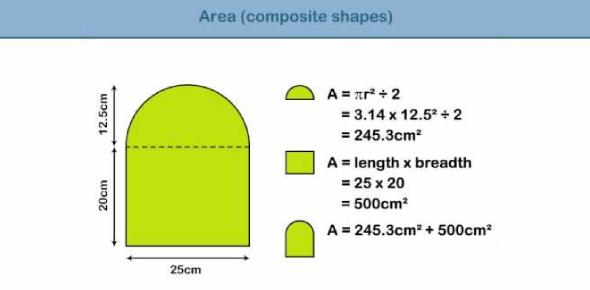Area Of Compound Shapes

12 Questions | Total Attempts: 6135SettingsAssesses and reinforces the student's understanding of area calculation of different basic shapes, and how to subdivide compound shapes in order to calculate total area.

• 1.
In square units, what is the area of the square?
• A.

8

• B.

16

• C.

44

• 2.
In square units, what is the area of the rectangle?
• 3.
In square units, what is the area of the triangle?
• A.

68

• B.

21

• C.

34

• 4.
In square units, what is the area of the triangle?
• 5.
In square units, what is the area of the triangle?
• A.

39

• B.

78

• C.

19

• 6.
In square units, what is the area of the parallelogram?
• 7.
In square units, what is the area of the trapezium?
• 8.
In square units, what is the area of the trapezium?
• A.

240

• B.

120

• C.

168

• 9.
In square units, what is the area of the compound shape?
• 10.
In square units, what is the area of the compound shape?
• 11.
In square units, what is the area of the compound shape?
• A.

114

• B.

21

• C.

135

• 12.
In square units, what is the area of the compound shape?
Related TopicsBack to top